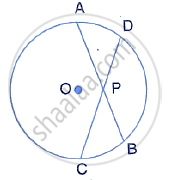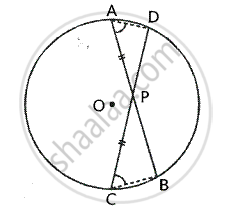Share

# Chords Ab and Cd of a Circle Intersect Each Other at Point P Such that Ap = Cp Show That: Ab = Cd - Mathematics

Course

#### Question

Chords AB and CD of a circle intersect each other at point P such that AP = CPShow that: AB = CD

#### SolutionGiven – two chords AB and CD intersect
Each other at P inside the circle
With centre O and AP = CP
To prove – AB = CD
Proof – Two chords AB and CD intersect each other inside the circle at P.
∴ AP × PB = CP× PD
⇒  (AP )/(CP)= (PD) / (PB)

But AP = CP    …….(1)          [given]

∴ PD = PB  or  PB = PD      ……. (2)